# A PSI4 Tutorial¶

## Basic Input File Structure¶

PSI4 reads input from a text file, which can be prepared in any standard text editor. The default input file name is input.dat and the default output file name is output.dat. So that you can give your files meaningful names, these defaults can be changed by specifying the input file name and output file name on the the command line. The syntax is:

>>> psi4 input-name output-name


## Running a Basic Hartree–Fock Calculation¶

In our first example, we will consider a Hartree–Fock SCF computation for the water molecule using a cc-pVDZ basis set. We will specify the geometry of our water molecule using a standard Z-matrix.

# Any line starting with the # character is a comment line
#! Sample HF/cc-pVDZ H2O computation

memory 250 mb

molecule h2o {
O
H 1 0.96
H 1 0.96 2 104.5
}

set basis cc-pVDZ
energy('scf')



For your convenience, this example can be found in tu1-h2o-energy. You can run it if you wish. Once PSI4 is in your path (see the User Configuration section of the installation instructions), you can run this computation by typing

>>> psi4 input.dat output.dat


If everything goes well, the computation should complete and should report a final restricted Hartree–Fock energy in a section like this:

Energy converged.

@RHF Final Energy:   -76.02665366589162

By default, the energy should be converged to about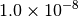, so agreement is only expected for about the first 8 digits after the decimal. If the computation does not complete, there is probably a problem with the compilation or installation of the program (see the installation instructions in Sec. Obtaining and Installing PSI4).

This very simple input is sufficient to run the requested information. Notice that we didn’t tell the program some otherwise useful information like the charge on the molecule (0, it’s neutral), the spin multiplicity (1 for a closed-shell molecule with all electrons paired), or the reference wavefunction to use (restricted Hartree–Fock, or RHF, is usually appropriate for a closed-shell molecule). The program correctly guessed all of these options for us. We can change the default behavior through additional keywords.

Let’s consider what we would do for an open-shell molecule, where not all electrons are paired. For example, let’s run a computation on methylene (CH2), whose ground electronic state has two unpaired electrons (triplet electronic state, or a spin multiplicity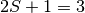). In this case, the default spin multiplicity (1) is not correct, so we need to tell the program the true value (3). Like many programs, PSI4 can get the charge and multiplicity as the first two integers in the Z-matrix. Note the line with 0 3 at the beginning of the molecule specification below. In this example we will also specify the bond length and bond angle as variables (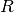and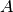), whose values are given at the end of the Z-matrix specification.

#! Sample UHF/6-31G** CH2 computation

memory 250 mb

molecule ch2 {
0 3
C
H 1 R
H 1 R 2 A

R = 1.075
A = 133.93
}

set basis 6-31G**
set reference uhf
energy ('scf')



This sample input can be found in tu2-ch2-energy and as before it can be run through the command psi4 input.dat output.dat (actually, because psi4 by default looks for an input file named input.dat and writes by default to a file called output.dat, in this case one could also just type psi4). If it works, it should print the final energy as

@UHF Final Energy:   -38.92534160932308

Notice we added a new keyword, set reference uhf, to the input. For open-shell molecules, we have a choice of unrestricted orbitals (unrestricted Hartree–Fock, or UHF), or restricted orbitals (restricted open-shell Hartree–Fock, or ROHF). Usually, UHF is a little easier to converge (although it may be more susceptible to spin contamination than ROHF).

## Geometry Optimization and Vibrational Frequency Analysis¶

The above examples were simple single-point energy computations (as specified by the energy() function). Of course there are other kinds of computations to perform, such as geometry optimizations and vibrational frequency computations. These can be specified by replacing energy() with optimize() or frequency(), respectively.

Here’s an example of optimizing the H2O molecule using Hartree–Fock with a cc-pVDZ basis set (located in tu3-h2o-opt.

#! Optimize H2O HF/cc-pVDZ

memory 250 mb

molecule h2o {
O
H 1 0.96
H 1 0.96 2 104.5
}

set basis cc-pVDZ
optimize('scf')



This should perform a series of gradient computations. The gradient points which way is downhill in energy, and the optimizer then modifies the geometry to follow the gradient. After about 4 cycles, the geometry should converge with a message like Optimization is complete!. As indicated in the following table (printed by the optimizer at the end of the computation), the energy decreases with each step, and the maximum force on each atom also decreases with each step (in principle these numbers could increase in some iterations, but here they do not).

----------------------------------------------------------------------
Step         Energy             Delta(E)      MAX force   MAX Delta(q)
----------------------------------------------------------------------
1    -76.026653665892    -76.026653665892    1.52e-02   1.52e-02
2    -76.026907793199     -0.000254127307    9.55e-03   9.55e-03
3    -76.027052927171     -0.000145133972    4.47e-04   4.47e-04
4    -76.027053472137     -0.000000544965    1.16e-04   1.16e-04
----------------------------------------------------------------------

To get harmonic vibrational frequencies, first we must set up an input using the OPTIMIZED GEOMETRY. We can easily get the optimized geometry from the previous computation. Looking at the output from running the previous example, we see that the OH bond length is about 0.9463 Ångstroms, and the bond angle is about 104.575 degrees. It’s good to give this many digits (or more) to make sure there’s not significant roundoff error in the geometry when running a frequency computation. So, our frequency computation input (which can be found as test case tu4-h2o-freq is:

#! Frequencies for H2O HF/cc-pVDZ at optimized geometry

memory 250 mb

molecule h2o {
O
H 1 0.9463
H 1 0.9463 2 104.575
}

set basis cc-pVDZ
frequencies('scf')



Alternatively, it’s also possible for PSI4 to use Cartesian coordinate input. Here, the Cartesian coordinates of the optimized geometry can be extracted from the bottom of the optimization output. The input would then look like this:

molecule h2o {
O     0.0000000000  -0.0000000000  -0.1224239500
H     0.0000000000  -1.4147069876   0.9714784639
H    -0.0000000000   1.4147069876   0.9714784639
}

set basis cc-pVDZ
frequencies('scf')

If either of the inputs above are run, the program should do some computations and then finally report the following harmonic vibrational frequencies (roundoff errors of around 0.1 cm-1 may exist):

  Irrep      Harmonic Frequency
(cm-1)
-----------------------------------------------
A1         1776.1735
A1         4113.8031
B2         4211.7879
-----------------------------------------------

Notice that the symmetry type of the normal modes is specified (A1, A1, B2). The program also prints out the normal modes in terms of Cartesian coordinates of each atom. For example, the normal mode at 1776 cm-1 is:

 Frequency:       1776.17
Force constant:   0.1194
X       Y       Z
O        0.000   0.000  -0.067
H        0.000   0.416   0.536
H        0.000  -0.416   0.536

where the table shows the displacements in the X, Y, and Z dimensions for each atom along the normal mode coordinate. (This information could be used to animate the vibrational frequency using visualization software.)

## Analysis of Intermolecular Interactions¶

Now let’s consider something a little more interesting. PSI4 contains code to analyze the nature of intermolecular interactions between two molecules, via symmetry-adapted perturbation theory (SAPT) [Jeziorski:1994:1887]. This kind of analysis gives a lot of insight into the nature of intermolecular interactions, and PSI4 makes these computations easier than ever.

For a SAPT computation, the input needs to provide information on two distinct molecules. This is very easy, we just give a Z-matrix or set of Cartesian coordinates for each molecule, and separate the two with two dashes, like this:

# Example SAPT computation for ethene*ethine (i.e., ethylene*acetylene),
# test case 16 from the S22 database

molecule dimer {
0 1
C   0.000000  -0.667578  -2.124659
C   0.000000   0.667578  -2.124659
H   0.923621  -1.232253  -2.126185
H  -0.923621  -1.232253  -2.126185
H  -0.923621   1.232253  -2.126185
H   0.923621   1.232253  -2.126185
--
0 1
C   0.000000   0.000000   2.900503
C   0.000000   0.000000   1.693240
H   0.000000   0.000000   0.627352
H   0.000000   0.000000   3.963929
units angstrom

no_reorient
symmetry c1
}

Notice we have a couple of new keywords in the molecule specification. no_reorient tells the program not to reorient the molecule to a different coordinate system (this is important for SAPT to make sure the same coordinate frame is used for the computations of the two monomers and for the dimer). The next keyword tells PSI4 to run in C1 point-group symmetry (i.e., without using symmetry), even if a higher symmetry is detected. SAPT computations know to turn off symmetry and fix orientation even without the user specifying these molecule keywords.

Here’s the second half of the input:

set globals {
basis jun-cc-pVDZ
scf_type DF
freeze_core True
}

energy('sapt0')

Before, we have been setting keywords individually with commands like set basis cc-pVDZ. Because we have a few more options now, it’s convenient to place them together into the set globals or set block, bounded by {...}. This will set all of these options as “global” options (meaning that they are visible to all parts of the program). Most common PSI4 options can be set in a globals section like this. If an option needs to be visible only to one part of the program (e.g., we only want to increase the energy convergence in the SCF code, but not the rest of the code), it can be placed in a section of input visible to that part of the program (e.g., set scf e_convergence 1.0E-8).

Back to our SAPT example, we have found that for basic-level SAPT computations (i.e., SAPT0), a good error cancellation is found [Hohenstein:2012:WIREs] with the jun-cc-pVDZ basis (this is the usual aug-cc-pVDZ basis, but without diffuse functions on hydrogen and without diffuse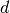functions on heavy atoms) [Papajak:2011:10]. So, we’ll use that as our standard basis set. The SAPT code is designed to use density fitting techniques, because they introduce minimal errors whil providing much faster computations [Hohenstein:2010:184111] [Hohenstein:2010:014101]. Since we’re using density fitting for the SAPT, we might as well also use it for the Hartree–Fock computations that are performed as part of the SAPT. We can specify that with scf_type DF.

Density fitting procedures require the use of auxiliary basis sets that pair with the primary basis set. Fortunately, PSI4 is usually smart enough to figure out what auxiliary basis sets are needed for a given computation. In this case, jun-cc-pVDZ is a standard enough basis set (just a simple truncation of the very popular aug-cc-pVDZ basis set) that PSI4 correctly guesses that we want the jun-cc-pVDZ-JKFIT auxiliary basis for the Hartree–Fock, and the jun-cc-pVDZ-RI basis set for the SAPT procedure.

To speed up the computation a little, we also tell the SAPT procedure to freeze the core electrons with freeze_core True. The SAPT procedure is invoked with the simple call, energy('sapt0')}. This call knows to automatically run two monomer computations and a dimer computation and then use these results to perform the SAPT analysis. The various energy components are printed at the end of the output, in addition to the total SAPT0 interaction energy. An explanation of the various energy components can be found in the review by Jeziorski, Moszynski, and Szalewicz [Jeziorski:1994:1887], and this is discussed in more detail in the SAPT section later in this manual. For now, we’ll note that most of the SAPT energy components are negative; this means those are attractive contributions (the zero of energy in a SAPT computation is defined as non-interacting monomers). The exchange contributions are positive (repulsive). In this example, the most attractive contribution between ethylene and acetylene is an electrostatic term of -2.12 kcal mol-1 (Elst10,r where the 1 indicates the first-order perturbation theory result with respect to the intermolecular interaction, and the 0 indicates zeroth-order with respect to intramolecular electron correlation). The next most attractive contribution is the Disp20 term (2nd order intermolecular dispersion, which looks like an MP2 in which one excitation is placed on each monomer), contributing an attraction of -1.21 kcal mol-1. It is not surprising that the electrostatic contribution is dominant, because the geometry chosen for this example has the acetylene perpendicular to the ethylene, with the acetylene hydrogen pointing directly at the double bond in ethylene; this will be attractive because the H atoms in acetylene bear a partial positive charge, while the electron-rich double bond in ethylene bears a partial negative charge. At the same time, the dispersion interaction should be smaller because the perpendicular geometry does not allow much overlap between the monomers. Hence, the SAPT analysis helps clarify (and quantify) our physical understanding about the interaction between the two monomers.

## Potential Surface Scans and Counterpoise Correction Made Easy with Psithon¶

Finally, let’s consider an example that shows how the Python driver in PSI4 simplifies some routine tasks. PSI4 can interpret valid Python code in addition to the computational chemistry directives we’ve seen in the previous examples; we call this mixture Psithon. The Python computer language is very easy to pick up, and even users previously unfamiliar with Python can use it to simplify tasks by modifying some of the example input files supplied with PSI4 in the psi4/samples directory.

Suppose you want to do a limited potential energy surface scan, such as computing the interaction energy between two neon atoms at various interatomic distances. One simple but unappealing way to do this is to create separate input files for each distance to be studied. But most of these input files are identical, except that the interatomic distance is different. Psithon lets you specify all this in a single input file, looping over the different distances with an array like this: Rvals=[2.5, 3.0, 4.0].

Let’s also suppose you want to do counterpoise (CP) corrected energies. Counterpoise correction involves computing the dimer energy and then subtracting out the energies of the two monomers, each evaluated in the dimer basis. Again, each of these computations could be run in a separate input file, but because counterpoise correction is a fairly standard procedure for intermolecular interactions, PSI4 knows about it and has a built-in routine to perform counterpoise correction. It only needs to know what method you want to do the counterpoise correction on (here, let’s consider CCSD(T)), and it needs to know what’s monomer A and what’s monomer B. This last issue of specifying the monomers separately was already dealt with in the previous SAPT example, where we saw that two dashes in the molecule block can be used to separate monomers.

So, we’re going to do counterpoise-corrected CCSD(T) energies for Ne2 at a series of different interatomic distances. And let’s print out a table of the interatomic distances we’ve considered, and the CP-corrected CCSD(T) interaction energies (in kcal mol-1) at each geometry. Doing all this in a single input is surprisingly easy in PSI4. Here’s the input (available as tu6-cp-ne2).

#! Example potential energy surface scan and CP-correction for Ne2

memory 250 mb

molecule dimer {
Ne
--
Ne 1 R
}

Rvals=[2.5, 3.0, 4.0]

set basis aug-cc-pVDZ
set freeze_core True

# Initialize a blank dictionary of counterpoise corrected energies
# (Need this for the syntax below to work)
ecp = {}

for R in Rvals:
dimer.R = R
ecp[R] = cp('ccsd(t)')

print "\n"
print "CP-corrected CCSD(T)/aug-cc-pVDZ interaction energies\n\n"
print "        R (Ang)         E_int (kcal/mol)             \n"
print "-----------------------------------------------------\n"
for R in Rvals:
e = ecp[R] * 627.5095
print "        %3.1f            %10.6f\n" % (R, e)



First, you can see the molecule block has a couple dashes to separate the monomers from each other. Also note we’ve used a Z-matrix to specify the geometry, and we’ve used a variable (R) as the interatomic distance. We have not specified the value of R in the molecule block like we normally would. That’s because we’re going to vary it during the scan across the potential energy surface. Below the molecule block, you can see the Rvals array specified. This is a Python array holding the interatomic distances we want to consider. In Python, arrays are surrounded by square brackets, and elements are separated by commas.

The next lines, set basis aug-cc-pVDZ and set freeze_core True, are familiar from previous test cases. Next comes a slightly unusual-looking line, ecp = {}. This is Python’s way of initializing a “dictionary.” We’re going to use this dictionary to store the counterpoise-corrected energies as they become available. A dictionary is like an array, but we can index it using strings or floating-point numbers instead of integers if we want. Here, we will index it using floating-point numbers, namely, the R values. This winds up being slightly more elegant than a regular array in later parts of the input file.

The next section, beginning with for R in Rvals:, loops over the interatomic distances, R, in our array Rvals. In Python, loops need to be indented, and each line in the loop has to be indented by the same amount. The first line in the loop, dimer.R = R, sets the Z-matrix variable R of the molecule called dimer to the R value extracted from the Rvals array. The next line, ecp[R] = cp('ccsd(t)'), computes the counterpoise-corrected CCSD(T) energy and places it in the ecp dictionary with R as the index. Note we didn’t need to specify ghost atoms, and we didn’t need to call the monomer and dimer computations separately. The built-in Psithon function cp() does it all for us, automatically.

And that’s it! The only remaining part of the example input is a little table of the different R values and the CP-corrected CCSD(T) energies, converted from atomic units (hartree) to kcal mol-1 by multiplying by the automatically-defined conversion factor psi_hartree2kcalmol, which is defined in Sec. Scratch Files and the ~/.psi4rc File. Notice the loop overto create the table looks just like the loop over R to run the different computations, and the CP-corrected energies ecp[R] are accessed the same way they were stored. The print line at the end just specifies some formatting for the printed table (first element is a floating point number 3 spaces wide with one digit after the decimal, and the second element is a floating point number 10 spaces wide with 6 digits after the decimal); the format strings are the same as in the C programming language. For tables more complicated than the simple one used here, Psithon has built-in support for tables (see the next section).

The following section goes over Psithon in much more detail, but hopefully this example already makes it clear that many complex tasks can be done very easily in PSI4